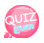# Aggregate Function

The aggregation function outputs the result of arithmetic operations such as the number of records, the sum of the values, the average, the maximum value, and the minimum value for the result records.
The following is a list of aggregate functions in MySQL.

Aggregate Function meaning
count(field name) the number of records that are not null
sum(field name) The sum of the values ​​of the field names
avg(field name) The average of the field name values
max(field name) The largest of the field names
main(field name) The smallest of the field names
count(*)
*means everything
Number of records

In order to learn the aggregation functions we will learn from now, we need to create a new table.
So let's make the table below

num name gender job area email rank
20200001 elsa w princess arendal elsa@quizgum.com 5
20200002 mickey m magician usa mickey@quizgum.com 7
20200003 minnie w disney character usa minnie@quizgum.com 2
20200004 rapunzel w princess usa rapunzel@quizgum.com 3
20200005 snow white w princess usa snow@quizgum.com 4
20200006 mike wazowski m Scarer usa wazo@quizgum.com 5
20200007 hiro m scientist usa bighero@quizgum.com 6
20200008 Yi Sun sin m general officer korea korea@quizgum.com 1
20200009 honda souichiro m engineer japan honda@quizgum.com 8
20200010 cinderella w princess usa bibidibabidiboo@quizgum.com 9

The database is called study_db and the table name is student.
So please create a database first.

`CREATE DATABASE study_db;`

So let's make a table. Please select a study_db.

`USE study_db;`

The following is a table creation statement as student:

```CREATE TABLE `student` (
`num` int(11) NOT NULL,
`name` varchar(10) DEFAULT NULL,
`gender` char(1) DEFAULT NULL,
`job` varchar(20) DEFAULT NULL,
`area` varchar(15) DEFAULT NULL,
`email` varchar(50) DEFAULT NULL,
`rank` int(11) DEFAULT NULL,
PRIMARY KEY (`num`)
) ENGINE=InnoDB DEFAULT CHARSET=utf8;```Now that we have a table, let's enter some data.

`INSERT INTO student VALUES(20120001, 'elsa', 'w', 'princess', 'arendal', 'elsa@quizgum.com', 5);`
`INSERT INTO student VALUES(20200002, 'mickey', 'm', 'magician', 'usa', 'mickey@quizgum.com', 7);`
`INSERT INTO student VALUES(20200003, 'minnie', 'w', 'disney character', 'usa', 'minnie@quizgum.com', 2);`
`INSERT INTO student VALUES(20200004, 'rapunzel', 'w', 'princess', 'usa', 'rapunzel@quizgum.com', 3);`
`INSERT INTO student VALUES(20120005, 'snow white', 'w', 'princess', 'usa', 'snow@quizgum.com', 4);`
`INSERT INTO student VALUES(20200006, 'mike wazowski', 'w', 'Scarer', 'usa', 'wazo@quizgum.com', 5);`
`INSERT INTO student VALUES(20200007, 'hiro', 'm', 'scientist', 'usa', 'bighero@quizgum.com', 6);`
`INSERT INTO student VALUES(20200008, 'Yi Sun sin', 'm', 'general officer', 'korea', 'korea@quizgum.com', 1);`
`INSERT INTO student VALUES(20200009, 'honda souichiro', 'm', 'engineer', 'japan', 'honda@quizgum.com', 8);`
`INSERT INTO student VALUES(20200010, 'cinderella', 'w', 'princess', 'usa', 'bibidibabidiboo@quizgum.com', 9);`

Let's enter the above statement.mike wazoski and honda souichiro got an error because the length of the string was longer than the value set in the name field. Increase the value of the name field further. Change varchar (10) to varchar (20).

```ALTER TABLE student CHANGE name name varchar(20);
DESC student;````SELECT * FROM student;`Okay ~~ Now let's study the aggregation function !!
Let's search the total number of students.
Enter the following command

`SELECT COUNT(*) FROM student;`Now let's get the ranking ranking of each region.
Group by area. Use a GROUP BY statement.

Group by area. Use a GROUP BY statement.

`SELECT area, avg(rank) FROM student GROUP BY area;`

In the above statement, the fields to be shown are area and rank.
We used the avg function to see the average, and the area averages of the rank values ​​are displayed.As shown above, each major was combined.
This time, let's search for a record with a specific string.
The operator used to search for records with a specific string is like, provided the characters '_' (underscore) and% (percent) to match the string constant.
_ Any single character (2 bytes for Korean, so use 2)
% Percent (any number of characters (including zero)
So let's find a record whose name starts with m.
This was done in record search ^^

`SELECT * FROM student WHERE name LIKE 'm%';`Let's use not like instead of like.

`SELECT * FROM student WHERE name NOT LIKE 'm%';`verything is searched except for names beginning with m ^^
This is also possible. Find people without an o at the end of their names

`SELECT * FROM student WHERE name NOT LIKE '%o';`This time, let's find someone whose name is 4 letters and whose end is o. Use _ (underbar) three times.

`SELECT * FROM student WHERE name LIKE '___o';`So this time, let's find out who has a name that starts with m.

`SELECT * FROM student WHERE area = 'usa' AND name LIKE 'm%';`In the next lesson, we will look at changing and deleting records.